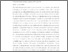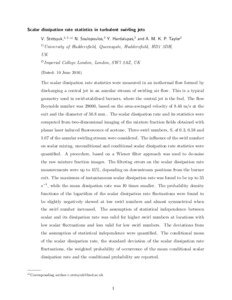Stetsyuk, V., Soulopoulos, N., Hardalupas, Y. and Taylor, A. M. K. P. (2016) Scalar dissipation rate statistics in turbulent swirling jets. Physics of Fluids, 28 (7). 075104. ISSN 1070-6631
Abstract

The scalar dissipation rate statistics were measured in an isothermal flow formed by discharging a central jet in an annular stream of swirling air flow. This is a typical geometry used in swirl-stabilised burners, where the central jet is the fuel. The flow Reynolds number was 29 000, based on the area-averaged velocity of 8.46 m/s at the exit and the diameter of 50.8 mm. The scalar dissipation rate and its statistics were computed from two-dimensional imaging of the mixture fraction fields obtained with planar laser induced fluorescence of acetone. Three swirl numbers, S, of 0.3, 0.58, and 1.07 of the annular swirling stream were considered. The influence of the swirl number on scalar mixing, unconditional, and conditional scalar dissipation rate statistics were quantified. A procedure, based on a Wiener filter approach, was used to de-noise the raw mixture fraction images. The filtering errors on the scalar dissipation rate measurements were up to 15%, depending on downstream positions from the burner exit. The maximum of instantaneous scalar dissipation rate was found to be up to 35 s−1, while the mean dissipation rate was 10 times smaller. The probability density functions of the logarithm of the scalar dissipation rate fluctuations were found to be slightly negatively skewed at low swirl numbers and almost symmetrical when the swirl number increased. The assumption of statistical independence between the scalar and its dissipation rate was valid for higher swirl numbers at locations with low scalar fluctuations and less valid for low swirl numbers. The deviations from the assumption of statistical independence were quantified. The conditional mean of the scalar dissipation rate, the standard deviation of the scalar dissipation rate fluctuations, the weighted probability of occurrence of the mean conditional scalar dissipation rate, and the conditional probability are reported.

Information
Library
DocumentsPreview
ArticleFile.pdf - Accepted Version